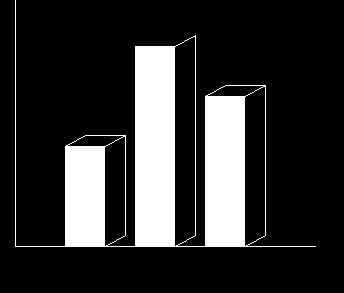# bar3d() function in C graphics

The header file graphics.h contains bar3d() function which is used to draw a 2-dimensional, rectangular filled in bar . Coordinates of left top and right bottom corner of bar are required to draw the bar.
Syntax :

```void bar3d(int left, int top, int right,
int bottom, int depth, int topflag);

where,
left specifies the X-coordinate of top left corner,
top specifies the Y-coordinate of top left corner,
right specifies the X-coordinate of right bottom corner,
bottom specifies the Y-coordinate of right bottom corner,
depth specifies the depth of bar in pixels,
topflag determines whether a 3 dimensional top is put on
the bar or not ( if it is non-zero then it is put otherwise not ).
```

Example :

```Input : left = 150, top = 250,
right = 190, bottom = 350,
depth = 20, topflag = 1

left = 220, top = 150,
right = 260, bottom = 350,
depth = 20, topflag = 0

left = 290, top = 200,
right = 330, bottom = 350,
depth = 20, topflag = 1
Output :```

Below is the implementation of bar3d() function :

 `// C Implementation for bar3d() function ` `#include ` ` `  `// driver code ` `int` `main() ` `{ ` `    ``// gm is Graphics mode which is ` `    ``// a computer display mode that ` `    ``// generates image using pixels. ` `    ``// DETECT is a macro defined in ` `    ``// "graphics.h" header file ` `    ``int` `gd = DETECT, gm; ` ` `  `    ``// initgraph initializes the ` `    ``// graphics system by loading a ` `    ``// graphics driver from disk ` `    ``initgraph(&gd, &gm, ``""``); ` ` `  `    ``// location of sides ` `    ``int` `left, top, right, bottom; ` ` `  `    ``// depth of the bar ` `    ``int` `depth; ` ` `  `    ``// top flag denotes top line. ` `    ``int` `topflag; ` ` `  `    ``// left, top, right, bottom, ` `    ``// depth, topflag location's ` `    ``bar3d(left = 150, top = 250, ` `    ``right = 190, bottom = 350, ` `    ``depth = 20, topflag = 1); ` ` `  `    ``bar3d(left = 220, top = 150, ` `    ``right = 260, bottom = 350, ` `    ``depth = 20, topflag = 0); ` ` `  `    ``bar3d(left = 290, top = 200, ` `    ``right = 330, bottom = 350, ` `    ``depth = 20, topflag = 1); ` ` `  `    ``// y axis line ` `    ``line(100, 50, 100, 350); ` ` `  `    ``// x axis line ` `    ``line(100, 350, 400, 350); ` ` `  `    ``getch(); ` ` `  `    ``// closegraph function closes the ` `    ``// graphics mode and deallocates ` `    ``// all memory allocated by ` `    ``// graphics system . ` `    ``closegraph(); ` ` `  `    ``return` `0; ` `} `

Output:

``````

Don’t stop now and take your learning to the next level. Learn all the important concepts of Data Structures and Algorithms with the help of the most trusted course: DSA Self Paced. Become industry ready at a student-friendly price.

My Personal Notes arrow_drop_upCheck out this Author's contributed articles.

If you like GeeksforGeeks and would like to contribute, you can also write an article using contribute.geeksforgeeks.org or mail your article to contribute@geeksforgeeks.org. See your article appearing on the GeeksforGeeks main page and help other Geeks.

Please Improve this article if you find anything incorrect by clicking on the "Improve Article" button below.

Article Tags :
Practice Tags :

Be the First to upvote.

Please write to us at contribute@geeksforgeeks.org to report any issue with the above content.